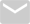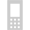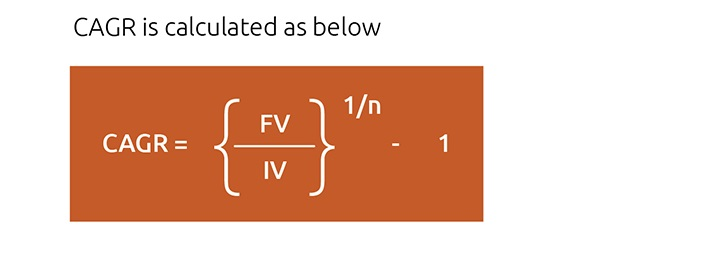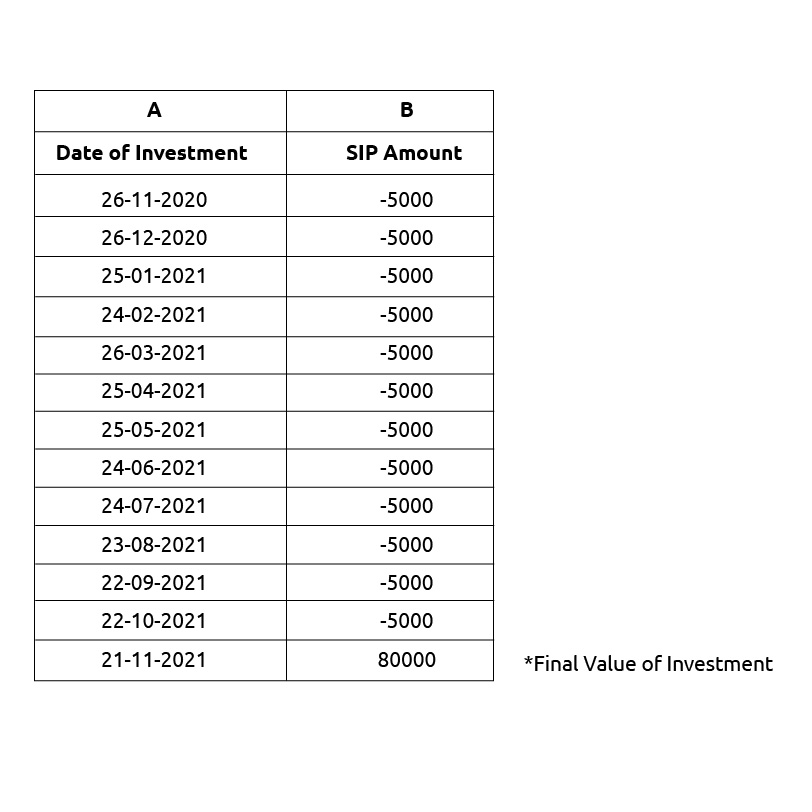Contact UsSMS EDU to 561617

# Financial Term of the week- Extended Internal Rate of Return (XIRR)We were taught very simply in primary school that the final price minus cost price equals profit and that the profit percentage is calculated on the cost price. But when we go deeper, we realize that this is no longer an effective measure of the returns we get from our financial investments. This is because in the above profit calculation, it is assumed that the amount invested, the period of investment and the returns remain constant. However, this may not be true.

There are different ways in which we can measure the returns from a mutual fund. First is the absolute return, which is nothing but the profit we mentioned above. If you invested Rs 5000 and got Rs 10,000 at the end of 5 years, your absolute return will be 100%. Second is the Compound Annual Growth Rate (CAGR); this is a more intricate measure that averages out the compounded returns of the investments every year to give you a representative figure of returns. So, the CAGR for the investment mentioned above is 14.87% (((10000/5000) ^1/5)-1).

Third, and the most effective measure of returns, especially in case of SIP in mutual funds, is XIRR. Let us understand why we need XIRR with an example. If you invested Rs 5000 for 12 months, let us assume the value of your investment at the end of 12 months is Rs 80,000. The reason why CAGR does not work here is that each amount is invested for a different period of time. The first Rs 5000 is invested for 12 months, the second Rs 5000 for 11 months, and so on. So, if you need an accurate return here, you will have to calculate the CAGR for every instalment and then sum them up. The XIRR does exactly that.

## How is XIRR calculated?

In simple words,

XIRR= Weighted average CAGR of all instalmentsIV= Investment Value (IV)

FV= Final Value (FV)

N= Investment intervals (n)

We understand it can be complicated to manually calculate XIRR for any investment. What’s more important is understanding its significance and need, especially when you are investing in mutual funds via SIP.

The simplest way of calculating XIRR is using Microsoft Excel, which uses an inbuilt formula to calculate it.

Taking the same example we quoted above-The formula is- XIRR (Values, Dates, Guess) = XIRR (B2:B14, A2:A14) = 67.91%

The last cell in the date column is the date on which you are calculating XIRR and the same in the value column is the maturity value of your investment on that particular date. We’d like to point out that the absolute return for the same investment would have been 33.33%. As you can see, XIRR provides you with a much more accurate and comprehensive measure for the returns earned.

## In conclusion-

You can make use of measures like CAGR, XIRR to understand if you are on the right investment path or not. In fact, not just for mutual funds alone, you can use this measure to calculate the return for any investment where the investment amount is not linear in nature.

Disclaimer:
Helpful information for investors: All Mutual Fund investors have to go through a one-time KYC (know your Customer) process. Investors should deal only with registered mutual funds, to be verified on SEBI website under 'Intermediaries/ Market Infrastructure Institutions'. For redressal of your complaints, you may please visit www.scores.gov.in . For more info on KYC, change in various details & redressal of complaints, visit mf.nipponindiaim.com/InvestorEducation/what-to-know-when-investing.htm This is an investor education and awareness initiative by Nippon India Mutual Fund.
MUTUAL FUND INVESTMENTS ARE SUBJECT TO MARKET RISKS, READ ALL SCHEME RELATED DOCUMENTS CAREFULLY.